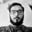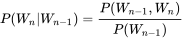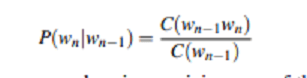Related Tags

machine learning
python
nlp
bigram
language

# What is a Bigram Language Model?Aman Anand

Language models are one of the most important parts of Natural Language Processing. In this shot, I will be implementing the simplest of the language models. The model implemented here is a “Statistical Language Model”. I have used “BIGRAMS” so this is known as the Bigram Language Model.

In the Bigram Language Model, we find bigrams, which are two words coming together in the corpus(the entire collection of words/sentences).

For example: In the sentence, Edpresso is awesome, and user-friendly the bigrams are :

• “Edpresso is”
• “is awesome”
• “and user”
• “user friendly”

In this code, the readData() function is taking four sentences that form the corpus. The sentences are:

• This is a dog
• This is a cat
• I love my cat
• This is my name

These sentences are split to find the atomic words that form the vocabulary.

Then, there is the function createBigram(), which finds all the possible Bigrams, dictionary of Bigrams, and Unigrams along with their frequency, i.e., how many times they occur in the corpus.

Then, the function calcBigramProb() is used to calculate the probability of each bigram. The formula for this is:It is in terms of probability we then use count to find the probability. Which is basically:We then use these probabilities to find the probability of the next word by using the chain rule, or we find the probability of the sentence as we have used it in this program. We will find the probability of the sentence, This is my cat in the program given below.

def readData():
data = ['This is a  dog','This is a cat','I love my cat','This is my name ']
dat=[]
for i in range(len(data)):
for word in data[i].split():
dat.append(word)
print(dat)
return dat

def createBigram(data):
listOfBigrams = []
bigramCounts = {}
unigramCounts = {}
for i in range(len(data)-1):
if i < len(data) - 1 and data[i+1].islower():

listOfBigrams.append((data[i], data[i + 1]))

if (data[i], data[i+1]) in bigramCounts:
bigramCounts[(data[i], data[i + 1])] += 1
else:
bigramCounts[(data[i], data[i + 1])] = 1

if data[i] in unigramCounts:
unigramCounts[data[i]] += 1
else:
unigramCounts[data[i]] = 1
return listOfBigrams, unigramCounts, bigramCounts

def calcBigramProb(listOfBigrams, unigramCounts, bigramCounts):
listOfProb = {}
for bigram in listOfBigrams:
word1 = bigram
word2 = bigram
listOfProb[bigram] = (bigramCounts.get(bigram))/(unigramCounts.get(word1))
return listOfProb

if __name__ == '__main__':
listOfBigrams, unigramCounts, bigramCounts = createBigram(data)

print("\n All the possible Bigrams are ")
print(listOfBigrams)

print("\n Bigrams along with their frequency ")
print(bigramCounts)

print("\n Unigrams along with their frequency ")
print(unigramCounts)

bigramProb = calcBigramProb(listOfBigrams, unigramCounts, bigramCounts)

print("\n Bigrams along with their probability ")
print(bigramProb)
inputList="This is my cat"
splt=inputList.split()
outputProb1 = 1
bilist=[]
bigrm=[]

for i in range(len(splt) - 1):
if i < len(splt) - 1:

bilist.append((splt[i], splt[i + 1]))

print("\n The bigrams in given sentence are ")
print(bilist)
for i in range(len(bilist)):
if bilist[i] in bigramProb:

outputProb1 *= bigramProb[bilist[i]]
else:

outputProb1 *= 0
print('\n' + 'Probablility of sentence \"This is my cat\" = ' + str(outputProb1))

The problem with this type of language model is that if we increase the n in n-grams it becomes computation-intensive. If we decrease the n, then long-term dependencies are not taken into consideration. Also, if an unknown word comes in the sentence, then the probability becomes 0. This problem of zero probability can be solved with a method known as smoothing. In smoothing, we also assign some probability to unknown words. Two very famous smoothing methods are:

• Laplace smoothing
• Good turing

RELATED TAGS

machine learning
python
nlp
bigram
language

CONTRIBUTORAman Anand